Function Repository Resource:

# TakeLarger

Take values larger than a threshold in a list or association

Contributed by: Wolfram staff
 ResourceFunction["TakeLarger"][list,val,n] gives the smallest n elements in list that are larger than val. ResourceFunction["TakeLarger"][list→prop,val,n] gives the property prop for the n elements in list larger than val. ResourceFunction["TakeLarger"][val,n] represents an operator form of ResourceFunction["TakeLarger"] that can be applied to an expression.

## Details and Options

ResourceFunction["TakeLarger"] sorts by numerical magnitude.
ResourceFunction["TakeLarger"] handles the same objects and properties as TakeLargest.
ResourceFunction["TakeLarger"] expects all objects to be comparable with one another.
ResourceFunction["TakeLarger"][list,val,UpTo[n]] takes n elements, or as many as are available.
ResourceFunction["TakeLarger"][assoc,val,n] gives an association of length n by taking the n smallest values that are larger than val in assoc, preserving their keys.
ResourceFunction["TakeLarger"][val,n][list] is equivalent to ResourceFunction["TakeLarger"][list,val,n].

## Examples

### Basic Examples (5)

Take two numbers larger than the specified value in a list:

 In:=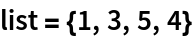Out=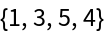In:=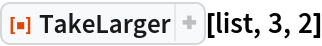Out=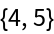Do the same using the operator form of TakeLarger:

 In:=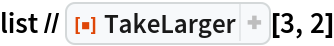Out=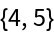In:=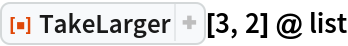Out=Take the smallest two values larger then the specified threshold in an association:

 In:=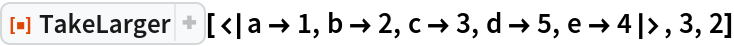Out=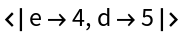Take the smallest four numbers larger than the specified value, or as many as are available:

 In:=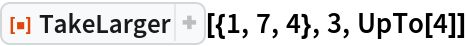Out=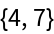Take all numbers larger than a threshold:

 In:=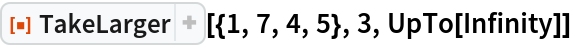Out=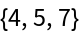Take two values in a list of Quantity objects:

 In:=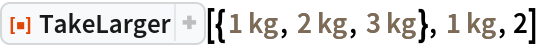Out=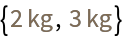### Scope (3)

Get the two smallest elements that are larger than 3 in a list:

 In:=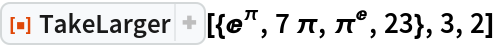Out=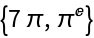Get the positions of the two smallest elements larger than 3:

 In:=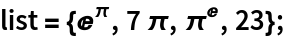In:=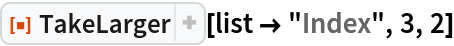Out=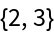Get the elements along with their positions:

 In:=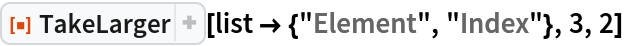Out=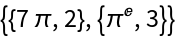Let positions come first in the result:

 In:=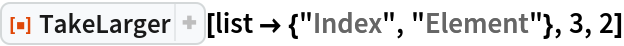Out=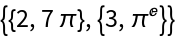Get associations containing the element and the position:

 In:=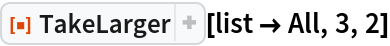Out=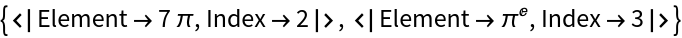Get the two smallest planets with the radii larger than the Earth:

 In:=Out=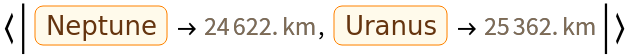## Version History

• 2.0.0 – 19 September 2019
• 1.0.0 – 08 July 2019